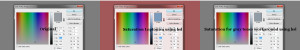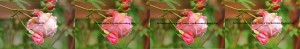# Leptonica saturation deficit workaround

While working with Leptonica I had the unpleasant surprise to notice that the saturation function pixModifySaturation was not really working properly (or at least not entirely as expected). First I didn’t even know what was wrong, I just felt it didn’t look right, then I saw that by transforming the saturation in Leptonica with pixModifySaturation , the contrast would be partially lost.Debugging the issue, and using different photos for the test some more problems have raised. The “almost” gray pixels(the gray colors have equal values for the red, green and blue, on the other hand these “almost” gray pixels are only approximately equal. eg: (34, 33, 38) ) within an image, using the same saturation function pixModifySaturation, have been saturated much more than the rest. In the photo with the pallet colors below you can notice the gray from the background that had a slight red tempt has turned a truly red color, also the gray with a slight tempt of blue from the current color box has turned a noticeable blue color.While almost all colors are correctly transformed, the downside of this issue can be noticed in real photos as “pixelated”, saturated areas(circled with red in the photo comparison below), looking very disturbing!The solution I’ve found, based on the fact that these “almost” gray pixels have been altered, was to make a sort of decreasing gradient from the given saturation factor for pixels that have a maximum difference value between red, green and blue, of a given threshold, let’s say 50.

```int max_diff_val = MAX(MAX(prv_rval,prv_gval),prv_bval)-MIN(MIN(prv_rval,prv_gval),prv_bval);
if(max_diff_val < 50)
fract_tmp = fract*max_diff_val/50;```

Even though it is known that a rgb(red, green, blue) to hsv(hue, saturation, value) pixel transformation works better for the saturation purpose, I liked more the contrast and luminosity given by the rgb to hsl(hue, saturation, luminosity) transformation. You can have a look at the comparison between the two (hsv vs hsl), in the photo below. You can choose the one that looks more appealing (click on the photo to maximize) :But Leptonica doesn't have a RGB to HSL function similar to the one for RGB to HSV(convertRGBToHSV), so I implemented the 2 needed functions: convertRGBToHSL and convertHSLToRGB for the conversion of rgb to hsl and the inverse one.
I've copied Leptonica's saturation function pixModifySaturation and made mypixModifySaturation transforming the code and adding the snippets mentioned above. You can see the entire code below. All the photos presented in this post have been transformed with a fract of 0.3 for the saturation(MypixModifySaturation(..,..,0.3)).

```PIX  *MypixModifySaturation(PIX       *pixd,
PIX       *pixs,
l_float32  fract)
{
l_int32    w, h, d, i, j, wpl;
l_int32    rval, gval, bval, hval, sval, lval, vval;
l_uint32  *data, *line;

PROCNAME("pixModifySaturation");

if (!pixs)
return (PIX *)ERROR_PTR("pixs not defined", procName, NULL);
pixGetDimensions(pixs, &w, &h, &d);
if (d != 32)
return (PIX *)ERROR_PTR("pixs not 32 bpp", procName, NULL);
if (L_ABS(fract) > 1.0)
return (PIX *)ERROR_PTR("fract not in [-1.0 ... 1.0]", procName, NULL);

pixd = pixCopy(pixd, pixs);
if (fract == 0.0) {
L_WARNING("no change requested in saturation", procName);
return pixd;
}

data = pixGetData(pixd);
wpl = pixGetWpl(pixd);
for (i = 0; i < h; i++) {
line = data + i * wpl;
for (j = 0; j < w; j++) {
extractRGBValues(line[j], &rval, &gval, &bval);
l_int32 prv_rval = rval,prv_gval=gval, prv_bval=bval;
//convertRGBToHSV(rval, gval, bval, &hval, &sval, &vval);
convertRGBToHSL(i,j,rval, gval, bval, &hval, &sval, &lval);
l_float32 fract_tmp = fract;
int max_diff_val = MAX(MAX(prv_rval,prv_gval),prv_bval)-MIN(MIN(prv_rval,prv_gval),prv_bval);
if(max_diff_val < 50)
{
fract_tmp = fract*max_diff_val/50;
}
if (fract_tmp < 0.0)
{
sval = (l_int32)(sval * (1.0 + fract_tmp));
}
else
{
sval = (l_int32)(sval + fract_tmp * (255 - sval));
}

//convertHSVToRGB(hval, sval, vval, &rval, &gval, &bval);
convertHSLToRGB(hval, sval, lval, &rval, &gval, &bval);
composeRGBPixel(rval, gval, bval, line + j);
}
}
return pixd;
}

void convertRGBToHSL
(int pixi, int pixj, l_int32 rval,l_int32 gval,l_int32 bval,
l_int32 *hval, l_int32 *sval, l_int32  *lval)
{
l_float32 r, g, b, h, s, l; //this function works with floats between 0 and 1

r = rval / 256.0;
g = gval / 256.0;
b = bval / 256.0;

/*Then, minColor and maxColor are defined. Mincolor is the value of the color component with the
smallest value, while maxColor is the value of the color component with the largest value.
These two variables are needed because the Lightness is defined as (minColor + maxColor) / 2.*/

float maxColor = MAX(r, MAX(g, b));
float minColor = MIN(r, MIN(g, b));

/*If minColor equals maxColor, we know that R=G=B and thus the color is a shade of gray.
This is a trivial case, hue can be set to anything, saturation has to be set to 0 because only
then it's a shade of gray, and lightness is set to R=G=B, the shade of the gray.*/

//R == G == B, so it's a shade of gray
if((r == g)&&(g == b))
{
h = 0.0; //it doesn't matter what value it has
s = 0.0;
l = r; //doesn't matter if you pick r, g, or b
}
/*If minColor is not equal to maxColor, we have a real color instead of a shade of gray,
so more calculations are needed:
Lightness (l) is now set to it's definition of (minColor + maxColor)/2.
nSaturation (s) is then calculated with a different formula depending if light is in the first
half of the second half. This is because the HSL model can be represented as a double cone, the
first cone has a black tip and corresponds to the first half of lightness values, the second cone
has a white tip and contains the second half of lightness values.
Hue (h) is calculated with a different formula depending on which of the 3 color components
is the dominating   one, and then normalized to a number between 0 and 1.*/
else
{

l = (minColor + maxColor) / 2;

if(l < 0.5) s = (maxColor - minColor) / (maxColor + minColor);
else s = (maxColor - minColor) / (2.0 - maxColor - minColor);

if(r == maxColor) h = (g - b) / (maxColor - minColor);
else if(g == maxColor) h = 2.0 + (b - r) / (maxColor - minColor);
else h = 4.0 + (r - g) / (maxColor - minColor);

h /= 6; //to bring it to a number between 0 and 1
if(h < 0) h ++;
}

/*Finally, H, S and L are calculated out of h,s and l as integers between 0 and 255 and
"returned" as the result. Returned, because H, S and L were passed by reference to the function.*/

*hval = int(h * 255.0);
*sval = int(s * 255.0);
*lval = int(l * 255.0);

}

void convertHSLToRGB
(l_int32 hval, l_int32 sval, l_int32 lval,
l_int32 *rval, l_int32 *gval, l_int32 *bval)
{
float r, g, b, h, s, l; //this function works with floats between 0 and 1
float temp1, temp2, tempr, tempg, tempb;
h = hval / 256.0;
s = sval / 256.0;
l = lval / 256.0;

/*Then follows a trivial case: if the saturation is 0, the color will be a grayscale color,
and the calculation is then very simple: r, g and b are all set to the lightness.*/

//If saturation is 0, the color is a shade of gray
if(s == 0)
{
r = l;
g = l;
b = l;
}
/*If the saturation is higher than 0, more calculations are needed again. red, green and blue
are calculated with the formulas defined in the code.*/

//If saturation > 0, more complex calculations are needed
else
{
//Set the temporary values
if(l < 0.5) temp2 = l * (1 + s);
else
temp2 = (l + s) - (l * s);
temp1 = 2 * l - temp2;
tempr = h + 1.0 / 3.0;
if(tempr > 1) tempr--;
tempg = h;
tempb = h - 1.0 / 3.0;
if(tempb < 0) tempb++;

//Red
if(tempr < 1.0 / 6.0) r = temp1 + (temp2 - temp1) * 6.0 * tempr;
else if(tempr < 0.5) r = temp2;
else if(tempr < 2.0 / 3.0) r = temp1 + (temp2 - temp1) * ((2.0 / 3.0) - tempr) * 6.0;
else r = temp1;

//Green
if(tempg < 1.0 / 6.0) g = temp1 + (temp2 - temp1) * 6.0 * tempg;
else if(tempg < 0.5) g = temp2;
else if(tempg < 2.0 / 3.0) g = temp1 + (temp2 - temp1) * ((2.0 / 3.0) - tempg) * 6.0;
else g = temp1;

//Blue
if(tempb < 1.0 / 6.0) b = temp1 + (temp2 - temp1) * 6.0 * tempb;
else if(tempb < 0.5) b = temp2;
else if(tempb < 2.0 / 3.0) b = temp1 + (temp2 - temp1) * ((2.0 / 3.0) - tempb) * 6.0;
else b = temp1;
}

//And finally, the results are returned as integers between 0 and 255.

*rval = int(r * 255.0);
*gval = int(g * 255.0);
*bval = int(b * 255.0);

}```